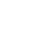Appendix A - Standard RenderMan Interface Shaders

In this section the required RenderMan Interface shaders are defined.

This shader does nothing and is intended to be a placeholder if no action is to be performed. There is a null shader for every class of shader.

surface
constant()
{
Oi = Os;
Ci = Os * Cs;
}

Matte surface

surface
matte(
float Ka = 1;
float Kd = 1;)
{
normal Nf = faceforward(N, I);
Oi = Os;
Ci = Os * Cs * (Ka*ambient() + Kd*diffuse(Nf));
}

Metal surface

surface
metal(
float Ka = 1;
float Ks = 1;
float roughness =.1;)
{
normal Nf = faceforward(N, I);
vector V = -normalize(I);
Oi = Os;
Ci = Os * Cs * (Ka*ambient()+Ks*specular(Nf, V, roughness));
}

Shiny metal surface

surface
shinymetal(
float Ka = 1;
float Ks = 1;
float Kr = 1;
float roughness = .1;
string texturename = ""; )
{
normal Nf = faceforward(N, I);
vector V = -normalize(I);
vector D = reflect(I, normalize(Nf));
D = vtransform("current", "world", D);
Oi = Os;
Ci = Os * Cs * (Ka*ambient() + Ks*specular(Nf, V, roughness)
+ Kr*color environment(texturename, D));
}

If the Environment Mapping capability is not supported by a particular renderer implementation, the shinymetal surface shader operates identically to the metal shader.

Plastic surface

surface
plastic(
float Ka = 1;
float Kd =.5;
float Ks =.5;
float roughness =.1;
color specularcolor = 1;)
{
normal Nf = faceforward(N, I);
vector V = -normalize(I);
Oi = Os;
Ci = Os * (Cs * (Ka*ambient() + Kd*diffuse(Nf)) +
specularcolor * Ks*specular(Nf, V, roughness) );
}

Painted plastic surface

surface
paintedplastic(
float Ka = 1;
float Kd = .5;
float Ks = .5;
float roughness = .1;
color specularcolor = 1;
string texturename = "";)
{
normal Nf = faceforward(N, I);
vector V = -normalize(I);
Oi = Os;
Ci = Os * (Cs * color texture(texturename) *
(Ka * ambient() + Kd * diffuse(Nf)) +
specularcolor * Ks * specular(Nf, V, roughness));
}

If the Texture Mapping capability is not supported by a particular renderer implementation, the paintedplastic surface shader operates identically to the plastic shader.

Ambient light source

light
ambientlight(
float intensity = 1;
color lightcolor = 1;)
{
Cl = intensity * lightcolor;
}

Distant light source

light
distantlight(
float intensity = 1;
color lightcolor = 1;
point from = point "shader" (0,0,0);
point to   = point "shader" (0,0,1);
{
solar(to-from, 0.0)
Cl = intensity * lightcolor;
}

Point light source

light
pointlight(
float intensity = 1;
color lightcolor = 1;
point from = point "shader" (0,0,0); )
{
illuminate(from)
Cl = intensity * lightcolor / L.L;
}

Spotlight source

light
spotlight(
float intensity = 1;
color lightcolor = 1;
point from = point "shader" (0,0,0);
point to = point "shader" (0,0,1);
float beamdistribution = 2;)
{
float atten, cosangle;
uniform vector A = (to - from)/length(to-from);
illuminate(from, A, coneangle) {
cosangle = L . A / length(L);
atten =  pow(cosangle, beamdistribution) / L.L;
atten *= smoothstep(cos(coneangle),
cos(coneangle - conedeltaangle), cosangle);
Cl = atten * intensity * lightcolor;
}
}

volume
depthcue(
float mindistance = 0, maxdistance = 1;
color background = 0;)
{
float d;
d = clamp((depth(P) - mindistance) /
(maxdistance - mindistance), 0.0, 1.0);
Ci = mix(Ci, background, d);
Oi = mix(Oi, color(1, 1, 1), d);
}

volume
fog(float distance = 1; color background = 0;)
{
float d;
d = 1 - exp(-length(I) / distance);
Ci = mix(Ci, background, d);
Oi = mix(Oi, color(1, 1, 1), d);
}

displacement
bumpy(
float Km = 1;
string texturename = "";)
{
float amp = Km * float texture(texturename, s, t);
P += amp * normalize(N);
N = calculatednormal(P);
}

If the Bump Mapping capability is not supported by a particular renderer implementation, the bumpy surface shader is equivalent to a null displacement shader.

imager
background( color background = 1; )
{
Ci += (1-alpha) * backaground;
Oi = 1;
alpha = 1;
}No part of this publication may be reproduced, stored in a retrieval system, or transmitted, in any form or by any means, electronic, mechanical, photocopying, recording, or otherwise, without the prior written permission of Pixar. The information in this publication is furnished for informational use only, is subject to change without notice and should not be construed as a commitment by Pixar. Pixar assumes no responsibility or liability for any errors or inaccuracies that may appear in this publication.